## Filters

Sort by :
Clear All
Q

10.(b)  What other name can you give to the line of symmetry of
(b) a circle?

The other name we can give to the line of symmetry of a circle is the diameter.

10.(a)  What other name can you give to the line of symmetry of
(a) an isosceles triangle?

The line of symmetry of an isosceles triangle is median or altitude.

9. Give three examples of shapes with no line of symmetry.

The  three examples of shapes with no line of symmetry are : 1. Quadrilateral  2. Scalene triangle  3.Parallelogram

8. What letters of the English alphabet have reflectional symmetry (i.e., symmetry related to mirror reflection)             about?
(a) a vertical mirror

(b) a horizontal mirror

(c) both horizontal and vertical mirrors

(a) a vertical mirror   : A,H,I,M,O,T,U,V,W,X and Y (b) horizontal mirror : B,C,D,E,H,I,O and X (c) both horizontal and vertical mirrors : H,I,O and X.

7.     State the number of lines of symmetry for the following figures:

(a) An equilateral triangle       (b) An isosceles triangle           (c) A scalene triangle
(d) A square                           (e) A rectangle                           (f) A rhombus
(g) A parallelogram                (h) A quadrilateral                      (i) A regular hexagon
(j) A circle

(a) An equilateral triangle         The number of lines of symmetry  = 3 (b) An isosceles triangle     The number of lines of symmetry  = 1          (c) A scalene triangle   The number of lines of symmetry  = 0  (d) A square      The number of lines of symmetry  = 4                            (e) A rectangle                             The number of lines of symmetry  = 2 (f) A rhombus  ...

6.   Copy the diagram and complete each shape to be symmetric about the mirror line(s):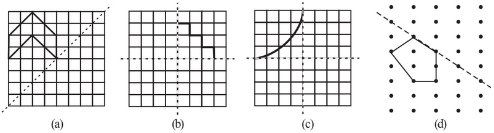The  complete  shape  symmetric about the mirror line(s) are :

5.    Copy the figure given here.
Take any one diagonal as a line of symmetry and shade a few more squares to make the figure symmetric about a diagonal. Is there more than one way to do that? Will the figure be symmetric about both the diagonals?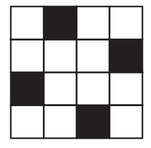The figure with symmetry may be as shown : Yes,there more than one way . Yes,the figure be symmetric about both the   diagonals

4.  The following figures have more than one line of symmetry. Such figures are said to have multiple lines of symmetry.Identify multiple lines of symmetry, if any, in each of the following figures: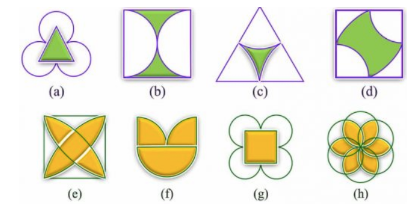The  lines of symmetry of figures are: (a)There are 3 lines of symmetry. Thus, it has multiple lines of symmetry. (b) There are 2 lines of symmetry. Thus, it has multiple lines of symmetry.   (c)There are 3 lines of symmetry. Thus, it has multiple lines of symmetry. (d)There are 2 lines of symmetry. Thus, it has multiple lines of symmetry. (e)There are 4 lines of symmetry. Thus, it has...

3.    In the following figures, the mirror line (i.e., the line of symmetry) is given as a dotted line. Complete each               figure performing reflection in the dotted (mirror) line. (You might perhaps place a mirror along the dotted line              and look into the mirror for the image). Are you able to recall the name of the figure you complete?The complete figures are as shown : (a)   square                (b)triangle            (c)rhombus               (d)circle                 (e) pentagon           (f) octagon

2. Given the line(s) of symmetry, find the other hole(s):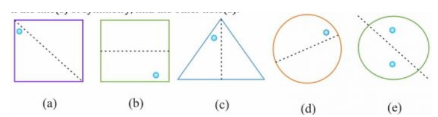The other holes  from the  symmetry are as shown :

1.(l) Copy the figures with punched holes and find the axes of symmetry for the following: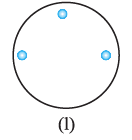The axes of symmetry is as shown :

1.(k) Copy the figures with punched holes and find the axes of symmetry for the following: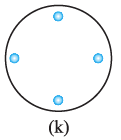The axes of symmetry is as shown :

1. Copy the figures with punched holes and find the axes of symmetry for the following: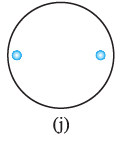The axes of symmetry is as shown :

1.(i)  Copy the figures with punched holes and find the axes of symmetry for the following: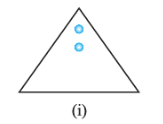The axes of symmetry is as shown :

1.(h) Copy the figures with punched holes and find the axes of symmetry for the following: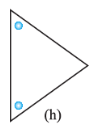The axes of symmetry are as shown :

1.(g) Copy the figures with punched holes and find the axes of symmetry for the following: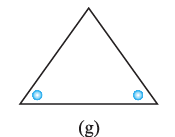The axes of symmetry is as shown :

1.(f)  Copy the figures with punched holes and find the axes of symmetry for the following: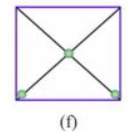The axes of symmetry is as shown :

1.(e) Copy the figures with punched holes and find the axes of symmetry for the following: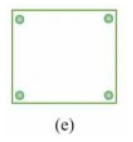The axes of symmetry is as shown :

1.(d) Copy the figures with punched holes and find the axes of symmetry for the following: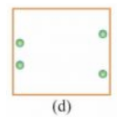The axes of symmetry is as shown :

1.(c)   Copy the figures with punched holes and find the axes of symmetry for the following: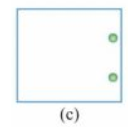The axes of symmetry is as shown :
Exams
Articles
Questions